﻿ Constant Stress Partially Accelerated Life Test Design for Inverted Weibull Distribution with Type-I CensoringAlgorithms Research

p-ISSN: 2324-9978    e-ISSN: 2324-996X

2013;  2(2): 43-49

doi:10.5923/j.algorithms.20130202.02

### Constant Stress Partially Accelerated Life Test Design for Inverted Weibull Distribution with Type-I Censoring

Mustafa Kamal, Shazia Zarrin, Arif-Ul-Islam

Department of Statistics & Operations Research, Aligarh Muslim University, Aligarh, 202002 (U.P.), India

Correspondence to: Mustafa Kamal, Department of Statistics & Operations Research, Aligarh Muslim University, Aligarh, 202002 (U.P.), India.
 Email: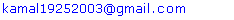Abstract

In Accelerated Life Testing, acceleration factor is known or there exists a mathematical model which specifies the life stress relationship. However, there are some situations in which neither acceleration factor is known nor such models exist or are very hard to assume. In such cases partially accelerated life tests are better criterion to use. This paper deals with simple Constant Stress Partially Accelerated life test using type-I censoring. Assuming that the lifetimes of test item follow Inverted Weibull distribution, the maximum likelihood estimation procedure is used to obtain the estimates for the distribution parameters and acceleration factor. Asymptotic confidence interval estimates of the model parameters are also evaluated by using Fisher information matrix. A Simulation study is used to illustrate the statistical properties of the parameters and the confidence intervals.

Keywords: Acceleration Factor, Maximum Likelihood Estimation, Reliability Function, Fisher Information Matrix, Asymptotic Confidence Interval, Simulation Study

Cite this paper: Mustafa Kamal, Shazia Zarrin, Arif-Ul-Islam, Constant Stress Partially Accelerated Life Test Design for Inverted Weibull Distribution with Type-I Censoring, Algorithms Research , Vol. 2 No. 2, 2013, pp. 43-49. doi: 10.5923/j.algorithms.20130202.02.

### 1. Introduction

In life testing and reliability experiments, time-to-failure data obtained under normal operating conditions is used to analyze the product’s failure-time distribution and its associated parameters. The continuous improvement in manufacturing design of today’s products and materials makes them highly reliable. Therefore, it is very difficult to obtain such life data while testing them at normal use conditions and the small time period between design and release. Under such conditions the life testing becomes very expensive and time consuming. These problems have motivated researchers to develop new life testing method and obtain timely information on the reliability of products, components and materials. Accelerated life testing (ALT) is then adopted and widely used in manufacturing industries. In ALT, products are tested at higher-than-usual levels of stress (e.g., temperature, voltage, humidity, vibration or pressure) to induce early failure. The life data collected from such accelerated tests is then analyzed and extrapolated to estimate the life characteristics under normal operating conditions by using a proper life stress relationship.
A lot of literature is available for ALT analysis. For example, Nelson[1, 2] provides a bibliography of 159 references on statistical plans for accelerated tests. Shan-rang et al. developed the statistical analysis method for obtaining estimators of the fouling life under usual stress level using type-II censored exponential life data under constant stress ALT models. Pascual presents methods for planning ALT in the presence of k competing risks. Ding et al. considers the design of ALT sampling plans under Type I progressive interval censoring with random removals. Tan et al. proposed Bayesian method for analyzing incomplete data obtained from constant stress ALT when there are two or more failure modes, or competing failure modes. Ding and Tse investigates the design of ALT plans under progressive Type II interval censoring with random removals assuming that the lifetimes of items follow a Weibull distribution, and the number of random removals at each inspection is assumed to follow a binomial distribution. Guo et al. present two methods for designing ALT to compare two different designs in terms of their B10 life. Balakrishnan and Han considered modification for censoring scheme in small sample sizes. Fan et al. discussed the maximum likelihood estimation (MLE) and Bayesian inference in group data ALT models under the relationship between the failure rate and the stress variables is linear under Box-Cox transformation. Al-Masri and Al-Haj Ebrahem derived the optimum times of changing stress level for simple step-stress plans under a cumulative exposure model assuming that the life time of a test unit follows a log-logistic distribution with known scale parameter by minimizing the asymptotic variance of the maximum likelihood estimator of the model parameters at the design stress with respect to the change time. Hassan and Al-Ghamdi obtained the optimal times of changing stress level for simple stress plans under a cumulative exposure model using the Lomax. Xu and Fei introduced and compared the four basic models for step-stress ALT: cumulative exposure model (CEM), linear cumulative exposure model (LCEM), tampered random variable model (TRVM), and tampered failure rate model (TFRM). Limitations of the four models were also discussed for better use of the models.
There are situations where a life stress relationship is not known and cannot be assumed, i.e., the data obtained from ALT cannot be extrapolated to use conditions. In such situations, partially accelerated life testing (PALT) is used. In PALT, test units are run at both use and accelerated conditions. Constant stress PALT and step stress are two commonly used methods. In constant stress PALT products are tested at either normal use or accelerated condition only until the test is terminated. In step stress PALT, a sample of test items is first run at use condition and, if it does not fail for a specified time, then it is run at accelerated condition until a prespecified numbers of failures are obtained or a prespecified time has reached.
So far a few research articles are available for constant stress PALT in which Bai & Chung consider constant stress PALT to obtain maximum likelihood estimates of the parameter of the exponential distribution and the acceleration under type-I censoring, Abdel-Ghaly et al. presented the MLE method to estimate the parameters of the Burr XII distribution with Types I and II censoring for a constant stress-PALT model, Abdel-Hamid also considered the MLE method to estimate the parameters of the Burr XII distribution with progressive Type II censoring for a constant stress-PALT model, Ali A. Ismail obtained the maximum likelihood estimators of the model parameters consider constant stress PALT with type-II censoring assuming that the lifetime at design stress has Weibull distribution. Cheng and Wang present a model for constant-stress PALT for Burr XII distribution under multiple censored data. The performance of the MLEs of the parameters was obtained by two maximization methods. One is quasi-Newton algorithm and other is theexpectation-maximization (EM) algorithm. More recently Zarrin et al. considered constant stress PALT with type-I censoring. Assuming Rayleigh distribution as the underlying lifetime distribution, the MLEs of the distribution parameter and acceleration factor were obtained.
In this paper Constant Stress PALT plan using type-I censoring assuming that the lifetimes of test item at use condition follow an Inverted Weibull distribution is considered. Maximum likelihood estimates and confidence intervals for parameters and acceleration factor are obtained. The performance of the inference method used in present papers is evaluated by a simulation study.

### 2. The Model

Notations: Some notations are used from Bai & Chung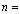total number of test items in a PALT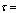censoring time of in constant stress PALT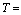life time of an item at use condition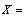life time of an item at accelerated condition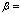acceleration factor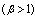and defined as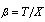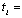observed life time of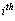item at use condition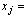observed life time of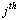item at accelerated condition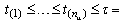ordered failure times at use condition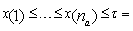ordered failure times at accelerated conditionThe Inverted Weibull distribution
The probability density function (PDF), cumulative distribution function (CDF), reliability function (RF) and hazard function (HF) for Inverted Weibull distribution with scale parameter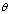and shape parameter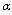are given respectively by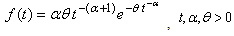(1)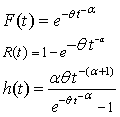Constant Stress PALT Procedure:
i. Total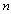items are divided randomly into two samples of sizes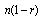and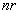respectively where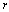is sample proportion. First sample is allocated to normal use condition and other is assigned to accelerated conditions.
ii. Each test item of every sample is run without changing the test condition until the censoring time.
Assumptions:
i. At use condition the lifetime of a test item follows an Inverted Weibull distribution given in (1).
ii. The lifetime of a test item at accelerated condition is obtained by using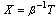where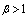, is an acceleration factor. Therefore, the PDF at accelerated condition is given, for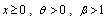,by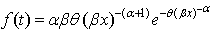(2)
iii. The lifetimes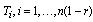of items allocated to normal condition are i.i.d. random variables.
iv. The lifetimes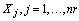, of items allocated to accelerated condition are i.i.d. random variables.
v. The lifetimes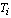and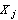are mutually independent.

### 3. Parameter Estimation

The maximum likelihood estimation method is used here because the ML method is very robust and gives the estimates of parameter with good statistical properties such as consistency, asymptotic unbiased, asymptotic efficiency and asymptotic normality. In this method, the estimates of parameters are those values which maximize the sampling distribution of data. However, ML estimation method is very simple for one parameter distributions but its implementation in ALT is mathematically more intense and, generally, estimates of parameters do not exist in closed form, therefore, numerical techniques such as Newton Method and some computer programs are used to compute them.
Let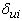and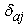be the indicator functions (Indicator Function is a function defined on a set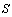that indicates membership of an element in a subset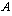of, having the value 1 for all elements ofand the value 0 for all elements ofnot in) at use and accelerated condition respectively, such that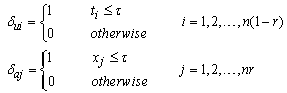(3)
The likelihood functions for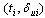and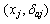are respectively given by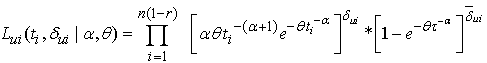(4)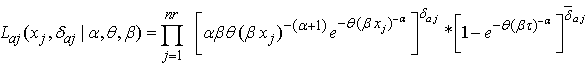(5)
where,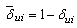and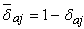.
The total likelihood for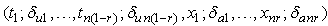is given by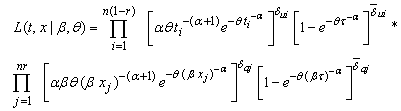(6)
The log likelihood function of (6) is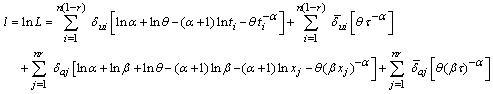(7)
MLEs of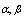and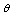are obtained by solving the system of equations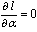,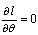and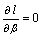respectively, where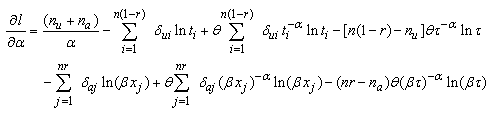(8)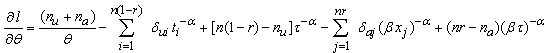(9)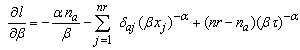(10)
where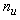represent the number of items failed at use condition and is given by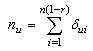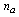represent number of items failed at accelerated condition and is given by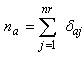Equations (8), (9) and (10) are non-linear equations and in order to obtain the MLEs of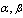and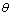, their solutions will numerically be obtained by using Newton Raphson method.

### 4. Asymptotic Confidence Interval

According to large sample theory, the maximum likelihood estimators, under some appropriate regularity conditions, are consistent and normally distributed. Since ML estimates of parameters are not in closed form, therefore, it is impossible to obtain the exact confidence intervals, so asymptotic confidence intervals based on the asymptotic normal distribution of ML estimators instead of exact confidence intervals are obtained here.
The observed Fisher information matrix can be expressed in terms of the second derivative of the log likelihood function under the regularity conditions as: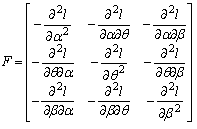where elements of Fisher information matrix are given as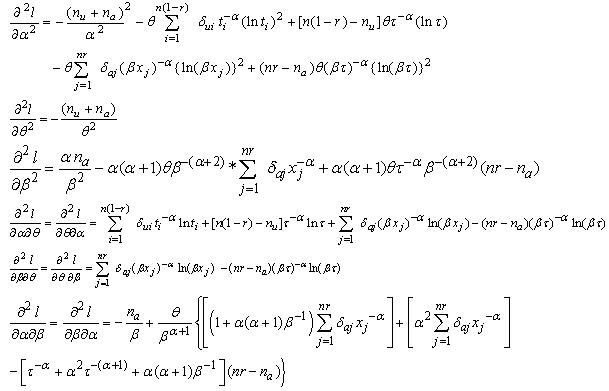Now, the asymptotic variance-covariance matrix of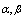andcan be written as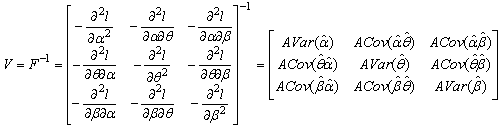The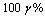asymptotic confidence interval for ofandare then given respectively by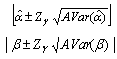and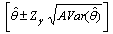### 5. Simulation Study

To evaluate the performance of the method of inference described in present study, first a random data with sample sizes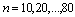and censoring times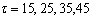is generated from Inverted Weibull distribution. The values of parameters are chosen to be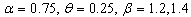. The estimators and the corresponding summary statistics are obtained by the present Constant Stress PALT model. For different given samples and censoring times with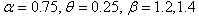the ML estimates of, the asymptotic standard error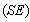the mean squared error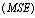and the coverage rate of the 95% confidence interval for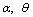and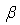based on 500 simulation replications are obtained and summarized in Table-1 and 2.
 Table 1. Simulation results for constant stress PALT with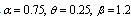and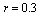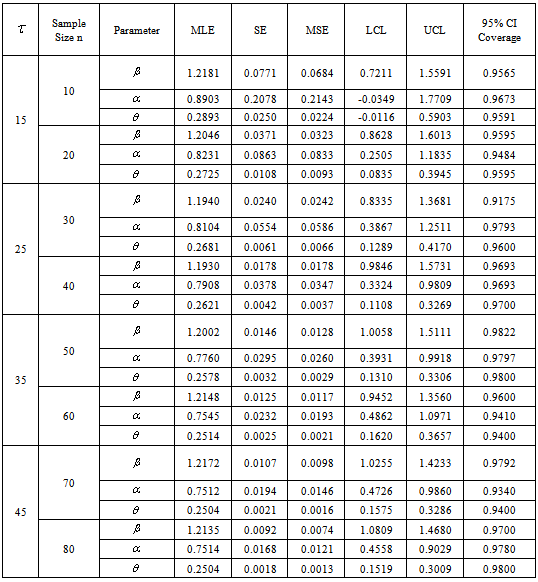Table 2. Simulation results for constant stress PALT with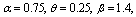and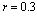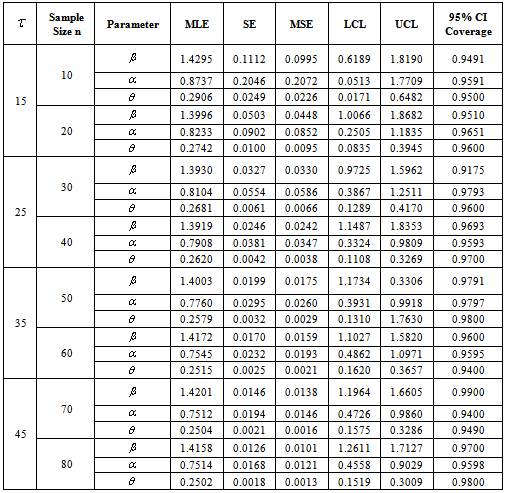### 6. Conclusions

From results in Table 1 and 2, it is observed that estimates obtained by the present model estimates the true parameters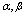andquite well respectively with relatively small mean squared errors. The estimated standard error also approximates well the sample standard deviation. For a fixedandwe find that as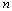increases, the mean squared errors of the estimates get smaller. This may be because that a larger sample size results in a better large sample approximation. It is also observed that the mean squared errors of the estimates get smaller as τ increases for a fixedand. It is also noticed that the coverage probabilities of the asymptotic confidence interval are close to the nominal level and do not change much across the different sample sizes. In short, it is reasonable to say that the present step stress ALT plan works well and has a promising potential in the analysis of accelerated life testing. For further future research in constant stress PALT one may consider some other life time distribution with different censoring schemes.

### References

  Nelson, W. (2005): A Bibliography of Accelerated Test Plans, Part I−Overview, IEEE Trans. on Reliability, vol. 54, pp. 194-197.  Nelson, W. (2005): A Bibliography of Accelerated Test Plans, Part II−References, IEEE Trans. on Reliability, vol. 54, pp. 370-373.  Shan-rang, Y., Ling-fang, S., Feng, G., Zhi-ming, X., Sheng-ping, W. (2006): Study on constant stress: Accelerated life tests of fouling thermal resistance. Heat Transfer-Asian Research, 35(2), pp. 110-114. doi:10.1002/htj.20105  Pascual, F. (2010): Accelerated life test planning with independent lognormal competing risks. Journal of Statistical Planning and Inference, 140(4), pp. 1089-1100. doi:10.1016/j.jspi.2009.11.003  Ding, C., Yang, C., Tse, S.K. (2010): Accelerated life test sampling plans for the Weibull distribution under Type I progressive interval censoring with random removals. Journal of Statistical Computation and Simulation, 80(8), pp. 903-914.  Tan, Y., Zhang, C., Chen, X. (2009): Bayesian analysis of incomplete data from accelerated life testing with competing failure modes. 2009 8th International Conference on Reliability, Maintainability and Safety, pp. 1268–1272. doi:10.1109/ICRMS.2009.5270049  Ding, C., and Tse, S.K. (2012): Design of accelerated life test plans under progressive Type II interval censoring with random removals. Journal of Statistical Computation and Simulation, 1–14. doi:10.1080/00949655.2012.660155  Guo, H., Niu, P., Mettas, A., & Ogden, D. (2012): On planning accelerated life tests for comparing two product designs, (RAMS), 2012. doi:10.1109/RAMS.2012.6175507  Balakrishnan, N. and Han, D. (2008): Optimal step-stress testing for progressively Type-I censored data from exponential distribution, Journal of Statistical Planning and Inference, vol. 138, pp. 4172-4186.  Fan T.H., Wang W.L. and Balakrishnan, N. (2008): Exponential progressive step-stress life testing with link function based on Box-Cox transformation, Journal of Statistical Planning and Inference, vol. 138, pp. 2340-2354.  Al-Masri, A.Q. and Al-Haj Ebrahem, M. (2009): Optimum Times for Step-Stress Cumulative Exposure Model Using Log-Logistic Distribution with Known Scale Parameter, Austrian Journal of Statistics, vol. 38, pp. 59-66.  Hassan, A. S. and Al-Ghamdi, A. S. (2009): Optimum Step Stress Accelerated Life Testing for Lomax Distribution, Journal of Applied Sciences Research, vol. 5, pp. 2153-2164.  Xu, H.Y. and Fei, H. (2012): Models Comparison for Step-Stress Accelerated Life Testing, Communications in Statistics - Theory and Methods, vol. 41, pp. 3878-3887.  Bai, D. S. and Chung, S. W. (1992), “Optimal Design of Partially Accelerated Life Tests for the Exponential Distribution under Type-I Censoring", IEEE Tran. on Reliability, Vol. 41 (3), pp 400-406. doi:10.1109/24.159807  Abdel-Ghaly, A. A., Amin, Z. H., Omar, D. A. (2008), Estimation of the Burr-XII distribution for partially accelerated life tests using censored data. Model Assisted Statistics and Applications, 3(4), pp 317–334.  Abdel-Hamid, A. H. (2009), Constant-partially accelerated life tests for Burr type-XII distribution with progressive type-II censoring. Computational Statistics & Data Analysis, Vol. 53(7), pp 2511–2523. doi:10.1016/j.csda.2009.01.018  Ismail, A. A. (2009), Optimum Constant-Stress Partially Accelerated Life Test Plans with Type-II Censoring: The Case of Weibull Failure Distribution, International Journal of Statistics & Economics, Vol. 3(S09), pp 39-46.  Cheng, Y. F. and Wang, F. K. (2012): Estimating the Burr XII Parameters in Constant-Stress Partially Accelerated Life Tests Under Multiple Censored Data, Communications in Statistics-Simulation and Computation, Vol. 41 (9), pp 1711-1727.  Zarrin, S., Kamal, M., Saxena, S. (2012) Estimation in Constant Stress Partially Accelerated Life Tests for Rayleigh Distribution Using Type-I Censoring, Reliability: Theory & Applications, Vol. 7 (4), pp 41-52.